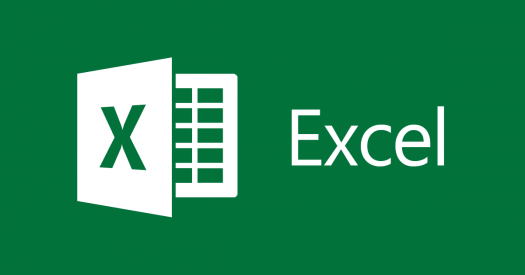# Basic Excel Trivia Quiz

13 Questions | Total Attempts: 609SettingsWhat we have here is a Basic Excel Trivia Quiz. Most people are not able to understand Microsoft excel on a higher level and that being said, some practice would come in handy, and the quiz below is one of many that will help you achieve that. Do give it a try and see how well you will do.

• 1.
You want to remove a formula from a cell and leave only the result. Which of the below is NOT a way to accomplish this?
• A.

Right Click and copy; then Right Click and Paste Special; select Values; OK

• B.

Ctrl + C; Ctrl + H, V, V

• C.

• D.

Copy; Paste

• 2.
• A.

=sumif

• B.

=sum

• C.

=

• D.

=sumif(

• 3.
A ________triangle appears in the upper-right corner of any cell with an attached comment.
• A.

Green triangle

• B.

Red triangle

• C.

Yellow triangle

• 4.
The format for an IF statement is:
• A.

=SUM(condition,x,y)

• B.

=IF(condition,x,y)

• C.

=(condition,x,y)

• 5.
A ______________ends the argument or formula.
• A.

Quotation mark

• B.

Closed parenthesis

• C.

Comma

• D.

Period

• 6.
To display a comment in a worksheet
• A.

Roll mouse over red triangle

• B.

Open comment cell

• C.

Must highlight cell

• 7.
If a range of cells is noted as \$A1:\$B2 in a formula, what will never change when copied to new cells?
• A.

The distance between A and B

• B.

The reference to column A and column B

• C.

The reference to row 1 and row 2

• D.

The reference to the entire range

• 8.
What is ALWAYS required in the range for a Pivot Table?
• A.

The range starting in cell A1

• B.

• C.

Numbers in all the columns

• 9.
The function SUMIFS adds numbers in a range of cells only if it matches the conditions you specify.
• A.

True

• B.

False

• 10.
___________ is a function that returns a number without a positive/negative sign designation.
• 11.
MID(text,start_num,num_chars)What does this function do?
• A.

Returns the middle number from a range of cells

• B.

Counts the number of characters in a text string

• C.

Converts all letters in a text string to lowercase

• D.

Returns the characters from the middle of a text string, with a specified starting position and length

• 12.
CONCATENATE(text1,text2...)What does this function do?
• A.

Joins several text strings into one

• B.

Evaluates whether the text strings are exactly the same

• C.

Removes all nonprintable characters from text

• D.

Replaces a text string with another

Related TopicsBack to top